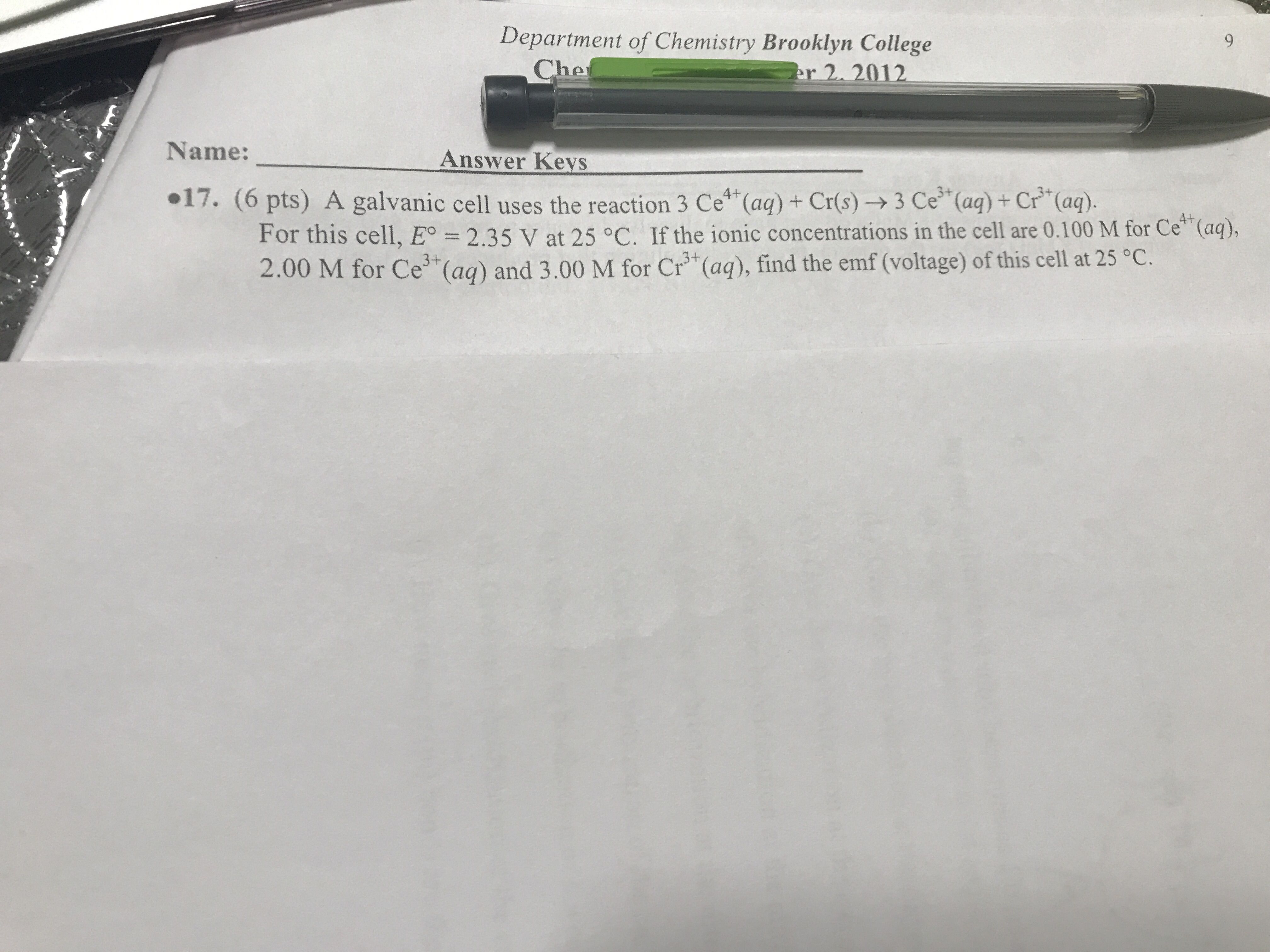# A galvanic cell uses the reaction 3 Ce^4+(aq) + Cr(s) ---> 3 Ce^3+(aq) + Cr3+(aq). For this cell, Eo = 2.35 V at 25 C. If the ionic concentrations in the cell are 0.100 M for Ce4+(aq), 2.00 M for Ce3+(aq) and 3.00 M for Cr3+(aq), find the emf (voltage) of this cell at 25 C